# Reparameterization: Tips and Tricks

This guide was originally written for scriptinghelpers. The original can be found here.

Here’s a guide about reparameterization. It has a few nifty tricks that can be applied to all sorts of things outside the topic.

Awhile back I wrote an article on the wiki discussing Bezier curves. Near the end of the article I started to talk about reparameterization (arc-length parameterization) and how it could be done without calculus. Today we are going to talk about how it can be done with calculus and hopefully make it clear how you might do this with functions beyond Bezier curves.

To start off let’s refresh ourselves on what I mean when I say reparameterization. Let’s say you are walking to your friend’s house across town. Your friend is quite impatient and at any given time will text you asking you how far along you are as a percentage. Now a sane person might look at a map and depending on their distance would provide a percentage, but what if there’s a mountain range between you and your friend, or the second half of the journey had a bullet train. These variables change how long different sections of the journey take and may vastly change our percentage estimation.

Mathematical functions sometimes face this exact problem and “slow down” or “speed up” at different sections. Reparameterization is the process of adjusting our mathematical functions such that when we plug in a percentage it returns a position relative to the total distance. In other words, it does not account for the “speed ups” and “slow downs”, 50% is half way the distance to the end no matter what.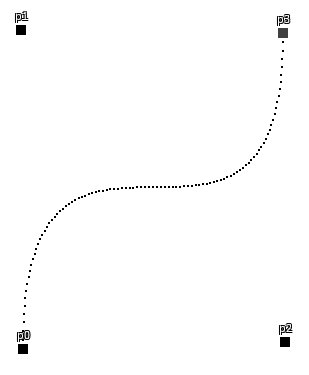Note: it slows down in the middle since the points are clustered together.

Right, so how do we reparameterize then? Typically, the process is as follows.

1. Use calculus to find the arc length of our function, `r(t)`. This should give us `s(t)` a function that will tell us the distance we have traveled along the curve for any percentage `t`.
2. Find the inverse of `s(t)` so that we can find the `t` that would be needed to achieve any given arc length, `t(s)`.
3. Use the inverse function to get reparametrized `t` value and plug in, `r(t(s))`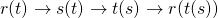So, using a quadratic Bezier as an example, step 1 is: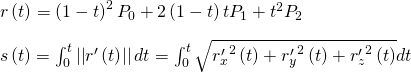Note that we are integrating the magnitude of the derivative of `r(t)`. Integration is essentially continuous summation. So think of this process as splitting the curve into infinitely small portions and then adding those up for the total length.

We could continue down this path and solve the integral which I have done here for your pleasure. Unfortunately, it is all for naught as solving for the integral gives us an `s(t)` where we can’t get the inverse function (or at least I couldn’t). Additionally, the math to solve it out is quite tough and does not generalize to other functions at all!

Our steps are good but tackling this problem head on isn’t. We have two issues that need solving:

1. How to integrate pretty much any function quickly and as accurately as possible.
2. How to find the `t` that would be needed to get a certain distance, `s`, along the curve.

# Simpson’s Rule

For our first problem let me introduce you to Simpson’s rule. It’s a technique that allows approximate integration by fitting a parabola to a function.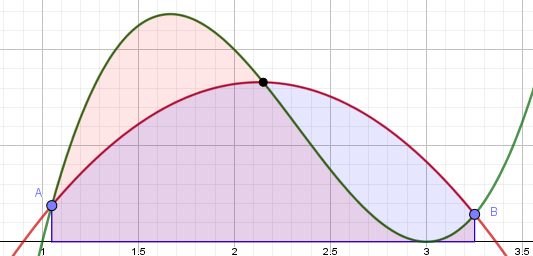In this case the green line is the function and the red line is the parabola that we are using to estimate the green’s definite integral between A and B. As you can hopefully see from the above picture this isn’t a very good estimation. However, if we adjust our A and B points to a smaller subsection our estimation becomes much more accurate!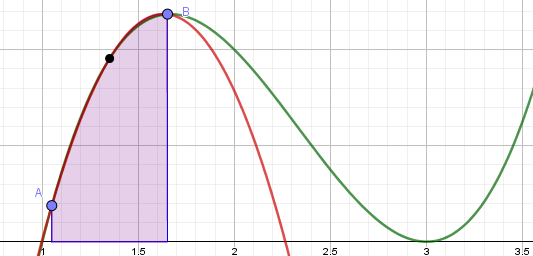We can do this multiple time with different intervals across the function, so we aren’t limited to small portions of the original function.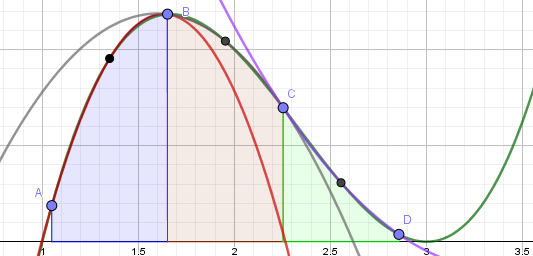So that partially answers our question. We can split up a function and use parabolas to estimate the integral. Now all we need to do is figure out how to actually do that calculation.

The first thing we need to recognize is that we’re trying to compute the area under the parabola, not the parabola itself. Thus, if we center our parabola around the origin of the graph we aren’t changing the area underneath it. Doing this step now makes algebra later much easier.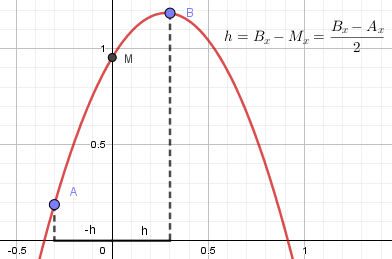We know the area under the above parabola can be represented by: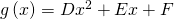Where D, E, and F are unknown constants. Thus, if we take the integral we get: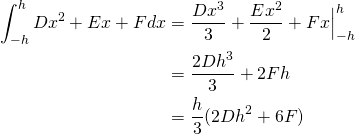We can easily figure out what `F` is with simple intuition. Since we centered the parabola around the origin we know that: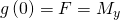As for figuring out D and E we know that: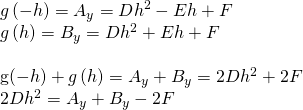Plugging that into our definite integral from above we get along side our F value.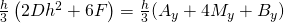Now recall, this only tends to be accurate for small intervals that a parabola can emulate well. To integrate larger areas, we must add a whole bunch of these smaller ones together.

Say we have some arbitrary function `f(x)` that we want to integrate using Simpson’s rule. By simply adding together a whole bunch of the above equation we can show that for any interval `x0` and `xn` if we have n subdivisions (where n is even) equally spaced apart we can approximate the integral.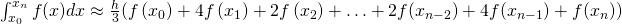Awesome we now have a way to get a good approximation of `s(t)` for pretty much any function.

We can write out the code and do some tests:

``````local function simpson(f, a, b, n)
if (n % 2 ~= 0) then
error("n must be even");
end
local h = (b - a) / n;
local s = f(a) + f(b);
for i = 1, n, 2 do
s = s + 4 * f(a + i * h);
end
for i = 2, n-1, 2 do
s = s + 2 * f(a + i * h);
end
return s * (h / 3);
end

local function BezierLength(p0, p1, p2)
-- the function we are integrating, ||r'(t)||, see the PDF posted earlier
local function arcLengthPrime(t)
local a = p0 - 2*p1 + p2;
local b = 2 * (p1 - p0);
local A = 4 * a:Dot(a);
local B = 4 * a:Dot(b);
local C = b:Dot(b);
return math.sqrt(A*t*t + B*t + C);
end

-- The higher n, the more accurate
local simpLength4 = simpson(arcLengthPrime, 0, 1, 4); -- 125.83485077414
local simpLength16 = simpson(arcLengthPrime, 0, 1, 16); -- 125.24746006847
local simpLength200 = simpson(arcLengthPrime, 0, 1, 200); -- 125.24747827975

-- I actually solved this integral so we can compare to the exact value (function in PDF)
local realLength = realBezierLength(1, p0, p1, p2);-- 125.24747828301

print(simpLength4, simpLength16, simpLength200, realLength);
end

local p0, p1, p2 = Vector3.new(0, 0, 0), Vector3.new(-15, 100, 20), Vector3.new(0, 40, -60);
BezierLength(p0, p1, p2);
``````

Sure enough Simpson’s rule seems to be quite accurate in telling us the length of the curve.

# Newton’s method

Okay, so that’s one of our problems solved, but we still need a way to get the t value that would correspond with a given distance, `d`. In other words, we need the t-value that solves the following:

`s(t) = d`

We can bring the d over to the other side and we’re left with a root finding problem:

`s(t) - d = 0`

This is problematic since if we try and tackle this problem head on we’re in essentially the same situation as before, we’d have to invert `s(t)`. However, now that we have a sure-fire way to estimate the value of `s(t)` we can use Newton’s root finding method.

Say we have an arbitrary function, `f(x)`, which has a real root. We aren’t exactly sure where the root is, but maybe we have a general idea (ex. The root is around 10 vs the root is around 1000). Something we can do to narrow our search is find a linear function that runs tangent to our estimate and then find the root of that. Using that newly found root we can repeat the process until we narrow in on the actual root of `f(x)`.

Visually: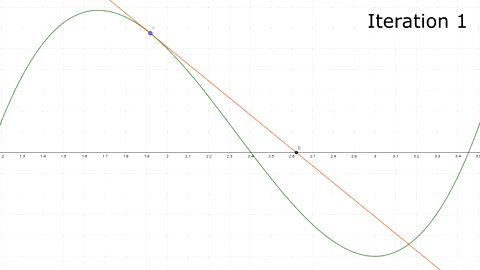So how can we mathematically compute this process? Say our best guess of the root is currently `xn`. We can find the slope of a line that runs tangent to that point by using the derivative `f'(xn)`. We also know that that the line has to pass through the point `(xn, f(xn))`. Using these pieces of information in addition to the standard form of a line we can solve for our line equation.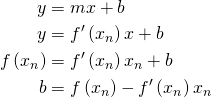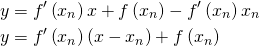Recall, the goal is to now find the root of the linear equation: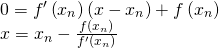Remember that this is an iterative method, so the x calculated here so then be used as the next guess and the process repeated: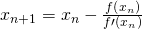So using the same example as in the above gif we can show how we can iterate to the root.

``````local function f(x)
return  (x - 3)^3 + 2*(x - 3)^2 - 0.5;
end

local function fp(x)
return 3*(x - 3)^2 + 4*(x - 3);
end

local xn = 2;
for i = 1, 6 do
xn = xn - (f(xn) / fp(xn));
print(xn);
end

-- results
> 2.5
> 2.4
> 2.4030303030303
> 2.4030317167624
> 2.4030317167627
> 2.4030317167627
``````

# Putting everything together

Now we have a simple method that we can use repeatedly to hone in on our root. In the case of reparameterization our equation will take the form: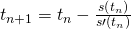We can calculate `s(t)` with Simpson’s rule, but what about `s'(t)`? Well that’s simply the function inside the `s(t)` integral, `||r'(t)||` (again, derived in the PDF).

Now that we have our two problems solved we can bring it all together:

``````local function quadBezier(t, p0, p1, p2)
return (1 - t)^2 * p0 + 2 * (1 - t) * t * p1 + t^2 * p2;
end

local function simpson(f, a, b, n)
if (n % 2 ~= 0) then
error("n must be even");
end
local h = (b - a) / n;
local s = f(a) + f(b);
for i = 1, n, 2 do
s = s + 4 * f(a + i * h);
end
for i = 2, n-1, 2 do
s = s + 2 * f(a + i * h);
end
return s * (h / 3);
end

local function reparamBezier(t, p0, p1, p2, n)
local function arcLengthPrime(t)
local a = p0 - 2*p1 + p2;
local b = 2 * (p1 - p0);
local A = 4 * a:Dot(a);
local B = 4 * a:Dot(b);
local C = b:Dot(b);
return math.sqrt(A*t*t + B*t + C);
end

local n = n or 16;
local length = simpson(arcLengthPrime, 0, 1, n);
-- find the distance we should travel ex. 50% of total distance
local d = t * length;

-- use newton's method to iterate to the t that would give the above distance
for i = 1, 5 do
t = t - (simpson(arcLengthPrime, 0, t, n) - d)/arcLengthPrime(t);
end

end

local p0, p1, p2 = Vector3.new(0, 0, 0), Vector3.new(0, 2, 0), Vector3.new(0, 10, 0);
print(quadBezier(0.5, p0, p1, p2)); -- 0, 3.5, 0 -> does not give the half way distance :(
print(reparamBezier(0.5, p0, p1, p2)); -- 0, 5, 0 -> Yay! 50% gives us 50% the distance!
``````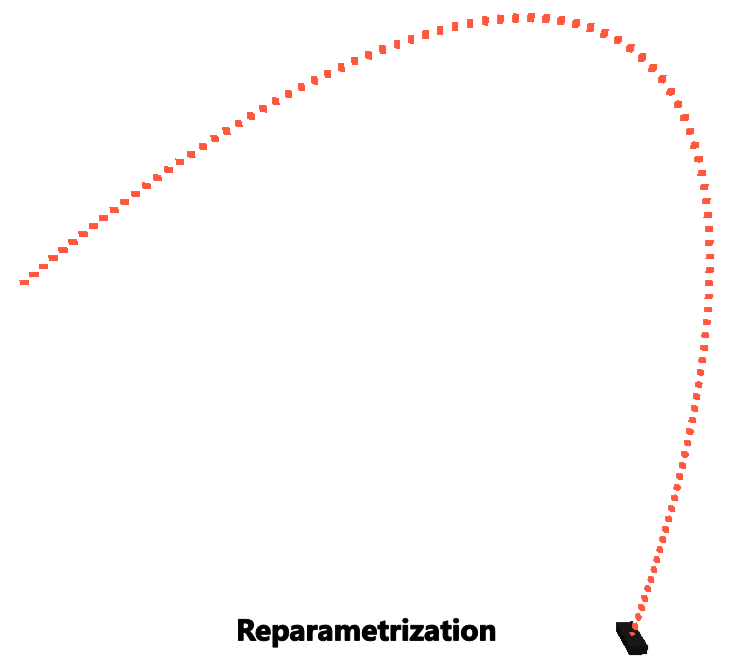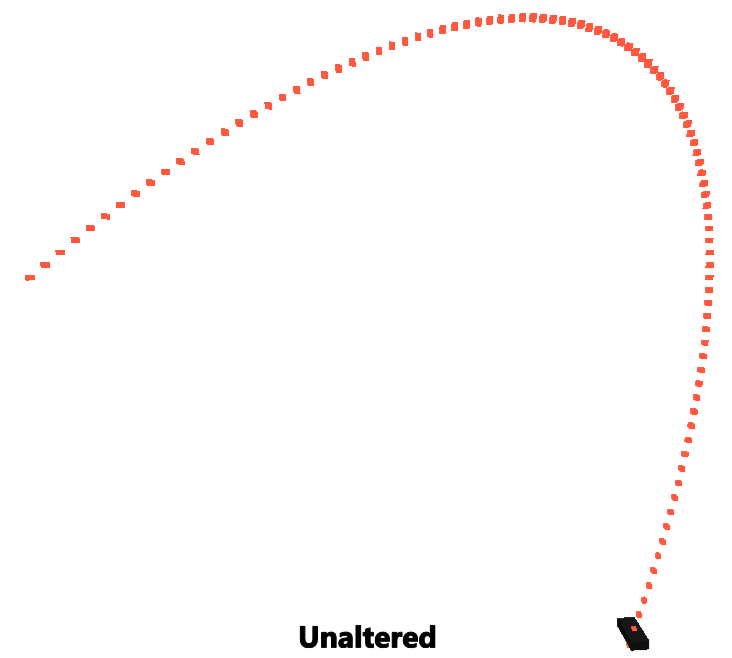# Conclusion

So that brings our journey to a close. Hopefully you learned some new techniques and tricks or at the very least new ways to apply them. Thanks for reading!

73 Likes

Sorry for the bump. However, if anyone else reading this needs a function for generating a reparameterized parabola then you can use this function:

``````local function parabola(t,p0,p1)
local g = Vector3.new(0, -game.Workspace.Gravity, 0);
return (0.5*g*t*t + (p1 - p0 - 0.5*g)*t + p0);
end

local function reparamParabola(t,p0, p1, n)
local function arcLengthPrime(t)
local a = (p1 - p0 - 0.5*Vector3.new(0, -game.Workspace.Gravity, 0)) - 2*p0;
local b = 2 * (p1 - p0);
local A = 4 * a:Dot(a);
local B = 4 * a:Dot(b);
local C = b:Dot(b);
return math.sqrt(A*t*t + B*t + C);
end

n = n or 16
local length = simpson(arcLengthPrime, 0, 1, n);
local d = t * length;

for i = 1, 5 do
t = t - (simpson(arcLengthPrime, 0, t, n) - d)/arcLengthPrime(t);
end

return parabola(t, p0, p1);
end
``````

EDIT: I have now realized that this will create points that are more evenly spaced the further down the line. If anyone has a solution for this please help.

2 Likes